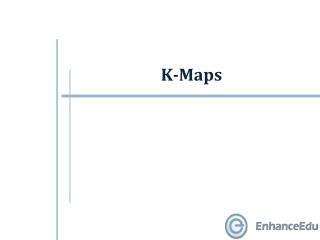DownloadDownload PresentationK-Maps

# K-Maps

Télécharger la présentation## K-Maps

- - - - - - - - - - - - - - - - - - - - - - - - - - - E N D - - - - - - - - - - - - - - - - - - - - - - - - - - -
##### Presentation Transcript

1. K-Maps

2. Outline • 2-variable K-maps • 3-variable K-maps • 4-variable K-maps • 5-variable and larger K-maps

3. Outline • 2-variable K-maps • 3-variable K-maps • 4-variable K-maps • 5-variable and larger K-maps

4. 2-variable K-maps (1/4) • Karnaugh-map (K-map) is an abstract form of Venn diagram, organised as a matrix of squares, where • each square represents a minterm • adjacent squares always differ by just one literal (so that the unifying theorem may apply: a + a' = 1) • For 2-variable case (e.g.: variables a,b), the map can be drawn as:

5. Alternative 1: Alternative 2: OR OR a a Alternative 3: a'b' ab' m0 m2 OR b a a b b ab m3 a'b m1 m0 m1 b ab a'b m3 m1 b a m3 m2 a'b' m0 ab' m2 b a'b' a'b a ab ab' 2-variable K-maps (2/4) • Alternative layouts of a 2-variable (a, b) K-map and others…

6. equivalent to: a a 1 0 b equivalent to: 0 1 b b 0 1 b a 0 1 a 2-variable K-maps (3/4) • Equivalent labeling:

7. C = a.b S = a.b' + a'.b b b 0 0 0 1 a a 1 0 0 1 2-variable K-maps (4/4) • The K-map for a function is specified by putting • a ‘1’ in the square corresponding to a minterm • a ‘0’ otherwise • For example: Carry and Sum of a half adder.

8. Outline • 2-variable K-maps • 3-variable K-maps • 4-variable K-maps • 5-variable and larger K-maps

9. b bc a 00 01 11 10 b 0 1 a'b'c' a'b'c a'bc a'bc' bc 00 01 11 10 a a ab'c' ab'c abc abc' 0 1 m0 m1 m3 m2 OR c a m4 m5 m7 m6 c Note Gray code sequence 3-variable K-maps (1/2) • There are 8 minterms for 3 variables (a, b, c). Therefore, there are 8 cells in a 3-variable K-map. Above arrangement ensures that minterms of adjacent cells differ by only ONE literal. (Other arrangements which satisfy this criterion may also be used.)

10. bc a 00 01 11 10 0 1 m0 m1 m3 m2 m4 m5 m7 m6 3-variable K-maps (2/2) • There is wrap-around in the K-map: • a'.b'.c' (m0) is adjacent to a'.b.c' (m2) • a.b'.c' (m4) is adjacent to a.b.c' (m6) Each cell in a 3-variable K-map has 3 adjacent neighbours. In general, each cell in an n-variable K-map has n adjacent neighbours. For example, m0 has 3 adjacent neighbours: m1, m2 and m4.

11. Outline • 2-variable K-maps • 3-variable K-maps • 4-variable K-maps • 5-variable and larger K-maps

12. y yz 00 01 11 10 wx 00 01 11 10 m0 m1 m3 m2 m4 m5 m7 m6 x m12 m13 m15 m14 w m8 m9 m11 m10 z 4-variable K-maps (1/2) • There are 16 cells in a 4-variable (w, x, y, z) K-map.

13. y yz wx m0 m1 m3 m2 m4 m5 m7 m6 x m12 m13 m15 m14 w m8 m9 m11 m10 z 4-variable K-maps (2/2) • There are 2 wrap-arounds: a horizontal wrap-around and a vertical wrap-around. • Every cell thus has 4 neighbours. For example, the cell corresponding to minterm m0 has neighbours m1, m2, m4 and m8.

14. Outline • 2-variable K-maps • 3-variable K-maps • 4-variable K-maps • 5-variable and larger K-maps

15. 5-variable K-maps (1/2) • Maps of more than 4 variables are more difficult to use because the geometry (hyper-cube configurations) for combining adjacent squares becomes more involved. • For 5 variables, e.g. vwxyz, need 25 = 32 squares.

16. v ' v y y yz yz 00 01 11 10 00 01 11 10 wx wx 00 01 11 10 m0 m1 m3 m2 00 01 11 10 m16 m17 m19 m18 m4 m5 m7 m6 m20 m21 m23 m22 x x m12 m13 m15 m14 m28 m29 m31 m30 w w m8 m9 m11 m10 m24 m25 m27 m26 z z 5-variable K-maps (2/2) • Organised as two 4-variable K-maps: Corresponding squares of each map are adjacent. Can visualise this as being one 4-variable map on TOP of the other 4-variable map.

17. Larger K-maps (1/2) • 6-variable K-map is pushing the limit of human “pattern-recognition” capability. • K-maps larger than 6 variables are practically unheard of! • Normally, a 6-variable K-map is organised as four 4-variable K-maps, which are mirrored along two axes.

18. b a'.b' a'.b ef 10 11 01 00 cd 00 01 11 10 m18 m19 m17 m16 ef 00 01 11 10 cd m22 m23 m21 m20 00 01 11 10 m0 m1 m3 m2 m30 m31 m29 m28 m4 m5 m7 m6 m26 m27 m25 m24 m12 m13 m15 m14 10 11 01 00 10 11 01 00 m58 m59 m57 m56 m8 m9 m11 m10 m40 m41 m43 m42 m62 m63 m61 m60 m44 m45 m47 m46 a m54 m55 m53 m52 m36 m37 m39 m38 m50 m51 m49 m48 m32 m33 m35 m34 cd 10 11 01 00 cd 00 01 11 10 ef a.b' a.b ef Larger K-maps (2/2) Try stretch your recognition capability by finding simplest sum-of-products expression for S m(6,8,14,18,23,25,27,29,41,45,57,61).